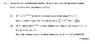# Normalising wavefunctions

• Lily Wright

## Homework Statement

2. Homework Equations [/B]
Uploaded as a picture as it's pretty hard to type out

## The Attempt at a Solution

So to normalise a wavefunction it has to equal 1 when squared.
A is the normalisation factor so we have:
A.x2e-x/2a0.x2e-x/2a0 = 1
∫ψ*ψdx = A2∫x4e-axdx = 1

Then I've been given the integral of this between 0 and ∞: ∫xne-axdx = n!/an+1
∫ψ*ψdx = A2.4!/a5
= A2.24/a5
A = √a5/24

Have I done this right? I don't even know where to start with (ii). Any help would be greatly appreciated!

#### Attachments

•physicalq1.jpg
20.6 KB · Views: 466